﻿ 基于无线通信的移动机器人视觉镇定控制

# 基于无线通信的移动机器人视觉镇定控制Vision Stabilization Control of Mobile Robot Based on Wireless Communication

Abstract: Based on wireless transmission technology, this paper designs a scheme for visual stabilization control of a wheeled mobile robot in a dynamic scene in response to an unknown target feature model. First, the coordinate system of the mobile robot’s visual servo system was established, and then the transformation relationship between the two desired coordinate systems before and after the feature point movement and the surveillance camera coordinate system was analyzed and calculated. The XBee-Pro wireless communication module is used to transmit feature point information. Simulation and experimental results show that the scheme can successfully drive the mobile robot to the target pose.

1. 引言

2. 系统坐标系描述

3. 控制策略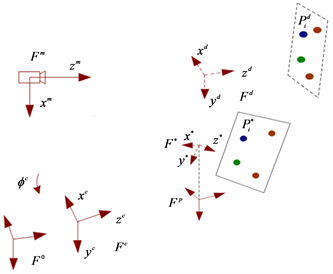Figure 1. Robot visual servoing system coordinate system

1) 第一阶段，机载摄像机在相对于目标特征点合理的位姿(特征点移动前期望位姿)处拍摄一张特征点移动之前的图像。然后移动机器人系统程序开始循环串口读取数据阶段，等待数据输入。接着固定监视摄像机拍取特征点移动之前的图像信息，运用XBee-Pro1发送。此时移动机器人系统无线通信模块XBee-Pro2接收XBee-Pro1发出的数据。机器人系统利用获取的数据经过六自由度单应矩阵分解得到 ${\mathcal{F}}^{m}$${\mathcal{F}}^{d}$ 之间的变换矩阵。

2) 第二阶段，特征点移动后，为完成从特征点移动后期望坐标系到原始坐标系的视觉镇定任务，令移动机器人在特征点移动后期望位姿出发。监视摄像机拍取特征点移动后的图像信息，利用模块XBee-Pro1将图像信息发出。然后机载相机拍取移动后特征点信息且XBee-Pro2接收XBee-Pro1发出的实时数据。最终经过六自由度矩阵分解得出 ${\mathcal{F}}^{m}$${\mathcal{F}}^{c}$ 间的变换矩阵。

3) 第三阶段，视觉伺服过程中，特征点的3D坐标是未知的，场景的深度信息亦不知，因此需要计算出两个不同深度信息之间的比例。然后经过坐标系变换，计算得出 ${\mathcal{F}}^{c}$${\mathcal{F}}^{d}$ 间的变换关系。

4) 第四阶段，运用自适应控制器驱使轮式移动机器人从特征点移动后期望位姿到达移动前原始期望位姿处。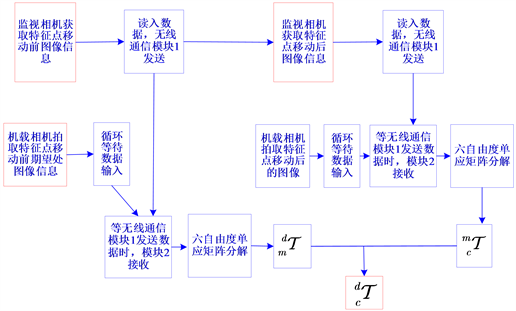Figure 2. Control strategy block diagram

4. 坐标系关系

${}_{m}{}^{d}R=\left[\begin{array}{ccc}{}_{m}{}^{d}R{}_{11}& {}_{m}{}^{d}R{}_{12}& {}_{m}{}^{d}R{}_{13}\\ {}_{m}{}^{d}R{}_{21}& {}_{m}{}^{d}R{}_{22}& {}_{m}{}^{d}R{}_{23}\\ {}_{m}{}^{d}R{}_{31}& {}_{m}{}^{d}R{}_{32}& {}_{m}{}^{d}R{}_{33}\end{array}\right],{}^{d}T{}_{m}=\left[\begin{array}{c}\frac{{}^{d}T{}_{mx}}{{d}^{m}}\\ \frac{{}^{d}T{}_{my}}{{d}^{m}}\\ \frac{{}^{d}T{}_{mz}}{{d}^{m}}\end{array}\right]$ (1)

${}_{m}{}^{c}R=\left[\begin{array}{ccc}{}_{m}{}^{c}R{}_{11}& {}_{m}{}^{c}R{}_{12}& {}_{m}{}^{c}R{}_{13}\\ {}_{m}{}^{c}R{}_{21}& {}_{m}{}^{c}R{}_{22}& {}_{m}{}^{c}R{}_{23}\\ {}_{m}{}^{c}R{}_{31}& {}_{m}{}^{c}R{}_{32}& {}_{m}{}^{c}R{}_{33}\end{array}\right],{}^{c}T{}_{m}=\left[\begin{array}{c}\frac{{}^{c}T{}_{mx}}{{d}^{mb}}\\ \frac{{}^{c}T{}_{my}}{{d}^{mb}}\\ \frac{{}^{c}T{}_{mz}}{{d}^{mb}}\end{array}\right]$ (2)

${}_{m}{}^{d}T=\left[\begin{array}{cc}{}_{m}{}^{d}R& \frac{{}^{d}T{}_{m}}{{d}^{m}}\\ {0}_{1×3}& 1\end{array}\right]$ (3)

${}_{m}{}^{c}T=\left[\begin{array}{cc}{}_{m}{}^{c}R& \frac{{}^{c}T{}_{m}}{{d}^{mb}}\\ {0}_{1×3}& 1\end{array}\right]$ (4)

${}_{m}{}^{c}T=\left[\begin{array}{cc}{}_{m}{}^{c}R& \frac{\lambda {}^{c}T{}_{m}}{{d}^{mb}}\\ {0}_{1×3}& 1\end{array}\right]=\left[\begin{array}{cc}{}_{m}{}^{c}R& \frac{{}^{c}T{}_{m}}{{d}^{m}}\\ {0}_{1×3}& 1\end{array}\right]$ (5)

$\left[\begin{array}{cc}{}_{d}{}^{c}R& \frac{{}^{c}T{}_{d}}{{d}^{m}}\\ {0}_{1×3}& 1\end{array}\right]=\left[\begin{array}{cc}{}_{m}{}^{c}R& \frac{{}^{c}T{}_{m}}{{d}^{m}}\\ {0}_{1×3}& 1\end{array}\right]{\left[\begin{array}{cc}{}_{m}{}^{d}R& \frac{{}^{d}T{}_{m}}{{d}^{m}}\\ {0}_{1×3}& 1\end{array}\right]}^{-1}$ (6)

$v=\left(\gamma \mathrm{cos}\alpha \right){e}_{s}$ (7)

$w=k\alpha +\stackrel{^}{l}\gamma \frac{\mathrm{cos}\alpha \mathrm{sin}\alpha }{\alpha }\left(\alpha +q\varphi \right)$ (8)

$\stackrel{˙}{\stackrel{^}{l}}=\Gamma \cdot {\text{Proj}}_{\stackrel{^}{l}}\left(\gamma \left(\alpha +q\varphi \right)\mathrm{sin}\alpha \mathrm{cos}\alpha \right)$ (9)

${\text{Proj}}_{\stackrel{^}{l}}\left(X\right)=\left\{\begin{array}{l}0,\text{\hspace{0.17em}}\text{\hspace{0.17em}}\text{ }\text{ }\stackrel{^}{l}={l}_{\mathrm{min}}\text{ }或\text{ }X<0\hfill \\ X,\text{\hspace{0.17em}}\text{\hspace{0.17em}}\text{ }其他\hfill \end{array}$ (10)

5. 仿真验证

$K=\left[\begin{array}{ccc}998.0& 0& 369.5\\ 0& 998.0& 369.5\\ 0& 0& 1\end{array}\right]$ (11)

$\left(-2.0\text{\hspace{0.17em}}m,1.0\text{\hspace{0.17em}}m,{0}^{\circ }\right)$ (12)

$\left(-2.3\text{\hspace{0.17em}}m,0.3\text{\hspace{0.17em}}m,{0}^{\circ }\right)$ (13)

$\gamma =1.38,k=1.0,q=2,{\stackrel{^}{l}}_{\mathrm{min}}=1$ (14)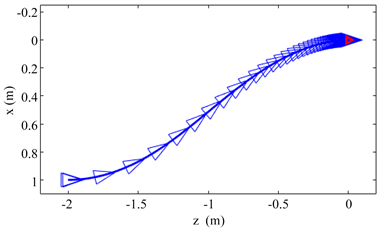Figure 3. Simulation results: wheeled robot movement path [Red triangle: desired pose]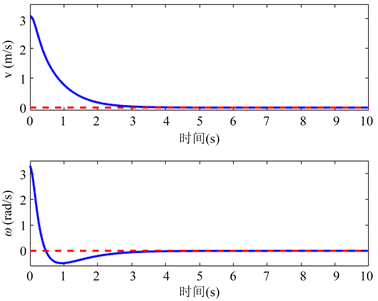Figure 4. Simulation results: speed change trend of the wheeled robot [Red dashed lines: desired values]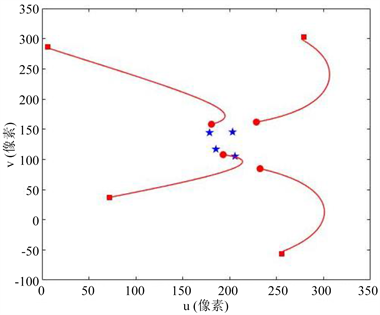Figure 5. Simulation results: target feature path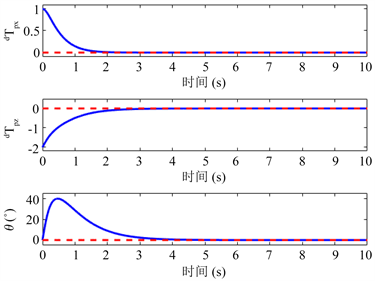Figure 6. Simulation results: evolution of the robot pose [Red dashed lines: desired values]

6. 实验验证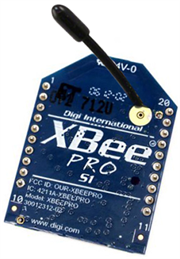Figure 7. XBee-Pro module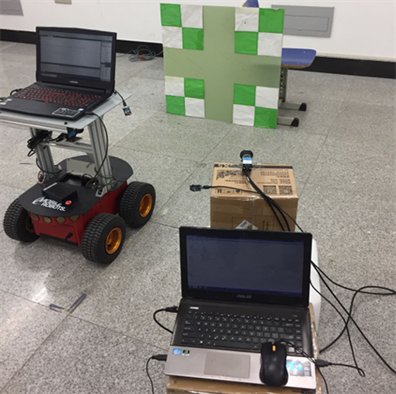Figure 8. Experiments scene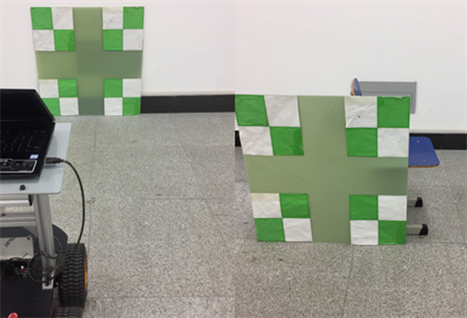Figure 9. Feature point movement [Left: before moving, Right: after moving]Figure 10. Experimental results: wheeled robot motion path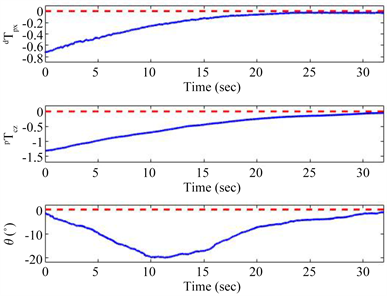Figure 11. Experimental results: evolution of the wheeled robot pose [Red dashed line: desired values]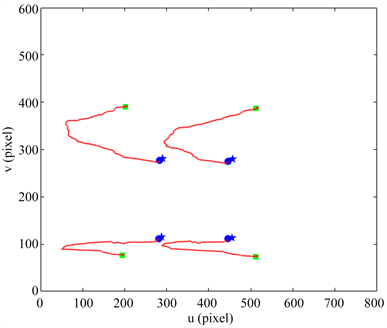Figure 12. Experimental results: feature point trajectory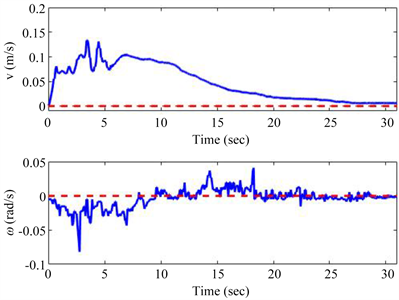Figure 13. Experimental results: speed change of wheeled robot [Red dashed line: zero values]

7. 结论

 谭民, 王硕. 机器人技术研究进展[J]. 自动化学报, 2013, 39(7): 963-972.

 Truong, X. and Ngo, T.D. (2017) Toward Socially Aware Robot Navigation in Dynamic and Crowded Environments: A Proactive Social Motion Model. IEEE Transactions on Automation Science and Engineering, 14, 1743-1760.
https://doi.org/10.1109/TASE.2017.2731371

 曹其新, 张蕾. 轮式自主移动机器人[M]. 上海: 上海交通大学出版社, 2012: 2.

 Hutchinson, S., Hager, G.D. and Corke, P.I. (1996) A Tutorial on Visual Servo Control. IEEE Transactions on Robotics and Automation, 12, 651-670.
https://doi.org/10.1109/70.538972

 Wang, H., Guo, D., Xu, H., et al. (2017) Eye-in-Hand Tracking Control of Free-Floating Space Manipulator. IEEE Transactions on Aerospace and Electronic Systems, 53, 1855-1865.
https://doi.org/10.1109/TAES.2017.2674218

 李宝全, 尹成浩, 师五喜. 模型未知动态场景下移动机器人的视觉镇定[J]. 天津工业大学学报, 2019, 38(3): 47-54.

 Yin, C., Li, B., Shi, W., et al. (2019) Monitoring-Based Visual Servoing of Wheeled Mobile Robots. International Journal of Robotics and Automation, 34, 230-330.
https://doi.org/10.2316/J.2019.206-0028

 Zhang, X., Fang, Y. and Liu, X. (2011) Motion-Estimation-Based Visual Servoing of Nonholonomic Mobile Robots. IEEE Transactions on Robotics, 27, 1167-1175.
https://doi.org/10.1109/TRO.2011.2162765

 Chen, J., Dixon, W.E., Dawson, D.M., et al. (2006) Homography-Based Visual Servo Tracking Control of a Wheeled Mobile Robot. IEEE Transactions on Robotics, 22, 406-415.
https://doi.org/10.1109/TRO.2006.862476

 陈华, 王朝立, 杨芳. 基于视觉伺服非完整移动机器人的有限时间饱和镇定[J]. 控制理论与应用, 2012, 29(6): 817-823.

Top